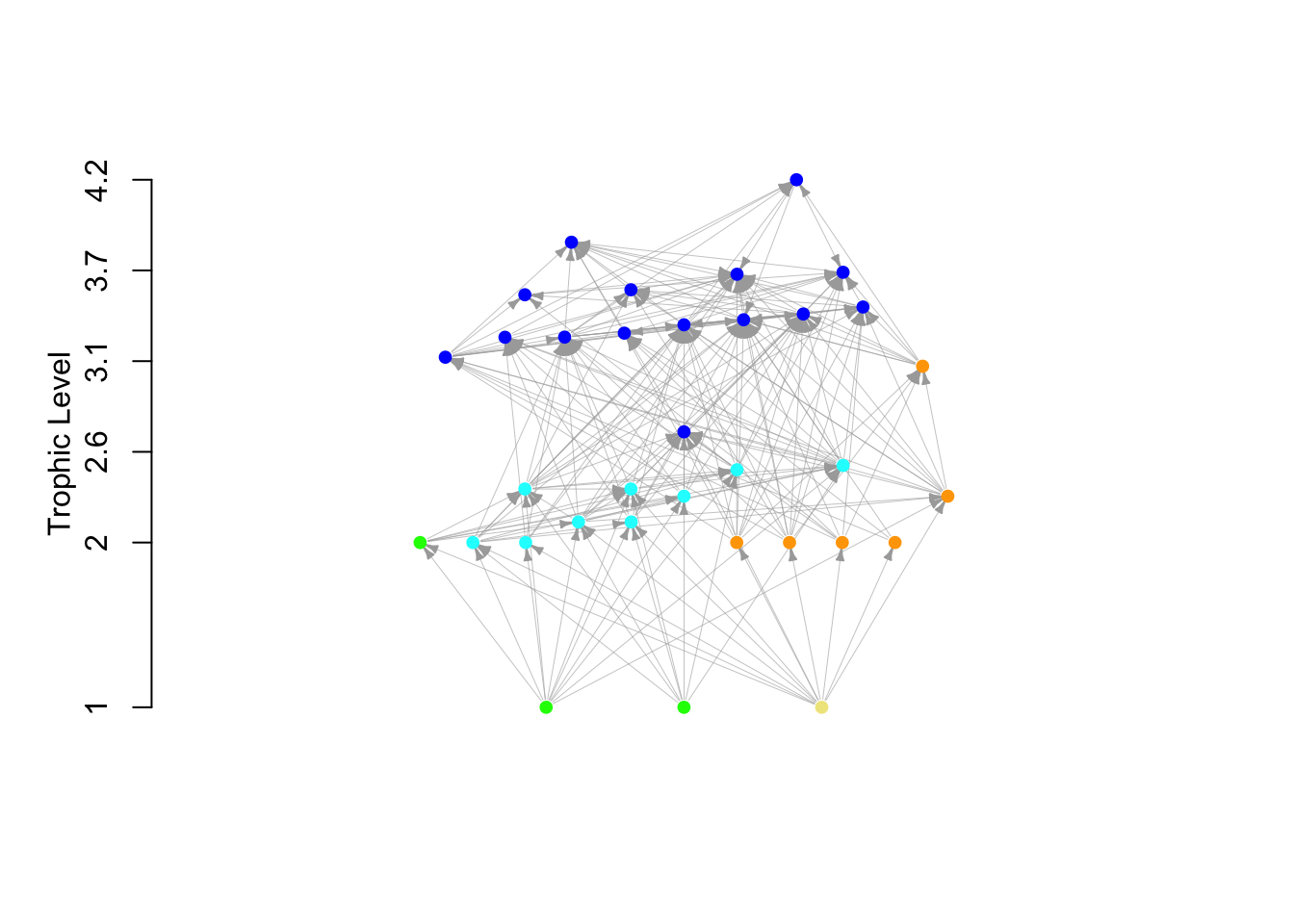This document provides an introduction to marine food web analyses. The tutorial targets students and scientists in marine biology and ecology with previous knowledge of the R software.

It is the companion tutorial for the article:
Kortsch, S., Frelat, R., Pecuchet, L., Olivier, P., Putnis, I., Bonsdorff, E., Ojaveer, H., Jurgensone, I., Strāķe, S., Rubene, G., Krūze, Ē., and Nordström, M. C. (2021). Disentangling temporal food web dynamics facilitates understanding of ecosystem functioning. Journal of Animal Ecology, 90(5), 1205-1216. DOI 10.1111/1365-2656.13447.

More details about data and methodology can be found in the Material and Methods section of the paper and in the supplementary material. For example, definitions and equations of metrics can be found in Appendix S6: Table S1. The raw food web dataset can be found on Dryad, DOI:10.5061/dryad.6t1g1jwwn.

Please also consult the tutorial by Ognyanova, K. (2016) Network analysis with R and igraph: NetSci X Tutorial for a detailed introduction to network analysis in R.

# Preliminaries

The analyses require the R packages igraph (v ≥ 1.2.4) and fluxweb (v ≥ 0.2.0).

``````library(igraph)
library(fluxweb)``````

If you get an error message, check that the R packages `igraph` and `fluxweb` have been installed correctly. If not, use the command: `install.packages(c("igraph", "fluxweb")`.

The metaweb food web of the Gulf of Riga is available as the Rdata file `BalticFW.Rdata`, available for download here.

Using the Gulf of Riga food web and this script, you will learn how to compute different weighted and unweighted network metrics to describe food webs.

# 1. Load and visualize the food web

Make sure the file `BalticFW.Rdata` is in your working directory, then load it in R.

``load("BalticFW.Rdata")``

The Rdata file contains two objects: `net` which is the `igraph` network of the Gulf of Riga food web, and `info` which is a `data.frame` containing information about each taxon. It also contains three custom-written functions that will be used in this script.

``vcount(net)``
``##  34``
``ecount(net)``
``##  207``

The metaweb `net` has 34 nodes and 207 trophic links.

``dim(info)``
``##  34 10``
``names(info)``
``````##   "species"      "fg"           "nbY"          "meanB"        "taxon"
##   "met.types"    "org.type"     "bodymasses"   "losses"       "efficiencies"``````

The data.frame `info` has 34 rows corresponding to the 34 nodes or taxa in the metaweb; and 10 variables which are:

• species: name of taxa
• fg: functional group of each taxa. There are 5 categories: Benthos, Fish, Phytoplankton, Zooplankton, and Detritus.
• nbY : number of year a taxa was recorded within the period 1979 - 2016
• meanB : average biomass for each taxon over the period 1979 - 2016
• taxon, met.types and org.types: classifications of taxa based on their taxonomy, metabolism type and organism type.
• bodymass: average body mass (in g) of an adult taxon.
• losses and efficiencies: metabolic parameters estimated using the `fluxweb` R package

## 1.2 Visualization of the food web

We will plot the food web using a custom-written function called `plotfw`, but first we will assign colors to the nodes depending on which functional group they belong to.

``````# See the functional group categories
levels(info\$fg)``````
``````##  "Benthos"       "Detritus"      "Fish"          "Phytoplankton"
##  "Zooplankton"``````
``````# Color the functional groups
colFG<- c("orange", "khaki", "blue", "green", "cyan")
# Assign the colors to each node (taxon)
info\$colfg <- colFG[as.numeric(info\$fg)]

# Plot the foodweb
# Nodes are colored (col) according to functional group
# Links (edge) size is reduced with edge.width and edge.arrow.size
plotfw(net, col=info\$colfg,
edge.width=0.3, edge.arrow.size=0.3)``````## 1.3 Overview of the food web

The function `degree()` returns the number of links per node (in-degree, out-degree or both). This is useful to quickly check the food web. For example, we can identify which are the basal species (no prey, i.e. 0 in-degree) and the top predators (no predators, i.e. 0 out-degree).

``````# basal species
V(net)\$name[degree(net, mode="in")==0]``````
``##  "Autotroph" "Mixotroph" "Detritus"``
``````# top predators
V(net)\$name[degree(net, mode="out")==0]``````
``##  "Gadus morhua"``

Three nodes classified as basal taxa: autotroph phytoplankon, mixotroph phytoplankton, and detritus. Only one species, cod (Gadus morhua), does not have any predators in our food web, and hence is the only top predator.

We can also plot the food web as an interaction matrix (called adjacency matrix), where the columns are the consumers and the rows the resources.

``````netmatrix <- get.adjacency(net, sparse=F)
heatmap(netmatrix, Rowv=NA, Colv=NA, scale="none")``````